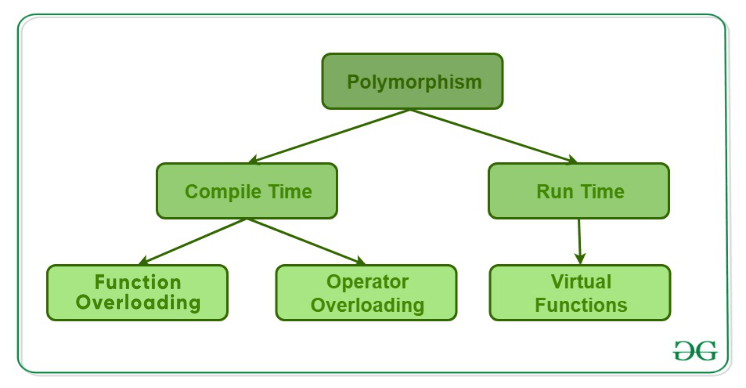Polymorphism in C++

• Difficulty Level : Easy
• Last Updated : 03 Jul, 2020

The word polymorphism means having many forms. In simple words, we can define polymorphism as the ability of a message to be displayed in more than one form. A real-life example of polymorphism, a person at the same time can have different characteristics. Like a man at the same time is a father, a husband, an employee. So the same person posses different behavior in different situations. This is called polymorphism. Polymorphism is considered as one of the important features of Object Oriented Programming.

In C++ polymorphism is mainly divided into two types:

• Compile time Polymorphism
• Runtime Polymorphism• Function Overloading: When there are multiple functions with same name but different parameters then these functions are said to be overloaded. Functions can be overloaded by change in number of arguments or/and change in type of arguments.
 // C++ program for function overloading#include   using namespace std;class Geeks{    public:          // function with 1 int parameter    void func(int x)    {        cout << "value of x is " << x << endl;    }          // function with same name but 1 double parameter    void func(double x)    {        cout << "value of x is " << x << endl;    }          // function with same name and 2 int parameters    void func(int x, int y)    {        cout << "value of x and y is " << x << ", " << y << endl;    }};  int main() {          Geeks obj1;          // Which function is called will depend on the parameters passed    // The first 'func' is called     obj1.func(7);          // The second 'func' is called    obj1.func(9.132);          // The third 'func' is called    obj1.func(85,64);    return 0;}

Output:

value of x is 7
value of x is 9.132
value of x and y is 85, 64

In the above example, a single function named func acts differently in three different situations which is the property of polymorphism.

• Operator Overloading: C++ also provide option to overload operators. For example, we can make the operator (‘+’) for string class to concatenate two strings. We know that this is the addition operator whose task is to add two operands. So a single operator ‘+’ when placed between integer operands , adds them and when placed between string operands, concatenates them.
Example:
 // CPP program to illustrate// Operator Overloading#includeusing namespace std;   class Complex {private:    int real, imag;public:    Complex(int r = 0, int i =0)  {real = r;   imag = i;}           // This is automatically called when '+' is used with    // between two Complex objects    Complex operator + (Complex const &obj) {         Complex res;         res.real = real + obj.real;         res.imag = imag + obj.imag;         return res;    }    void print() { cout << real << " + i" << imag << endl; }};   int main(){    Complex c1(10, 5), c2(2, 4);    Complex c3 = c1 + c2; // An example call to "operator+"    c3.print();}

Output:

12 + i9

In the above example the operator ‘+’ is overloaded. The operator ‘+’ is an addition operator and can add two numbers(integers or floating point) but here the operator is made to perform addition of two imaginary or complex numbers. To learn operator overloading in details visit this link.

2. Runtime polymorphism: This type of polymorphism is achieved by Function Overriding.
• Function overriding on the other hand occurs when a derived class has a definition for one of the member functions of the base class. That base function is said to be overridden.
 // C++ program for function overriding  #include using namespace std;  class base{public:    virtual void print ()    { cout<< "print base class" <print();            // Non-virtual function, binded at compile time    bptr->show();       return 0;}

Output:

print derived class
show base class

To learn runtime polymorphism in details visit this link.

This article is contributed by Harsh Agarwal. If you like GeeksforGeeks and would like to contribute, you can also write an article using contribute.geeksforgeeks.org or mail your article to contribute@geeksforgeeks.org. See your article appearing on the GeeksforGeeks main page and help other Geeks.